# Howdy Neighbor! Proximity Analysis in R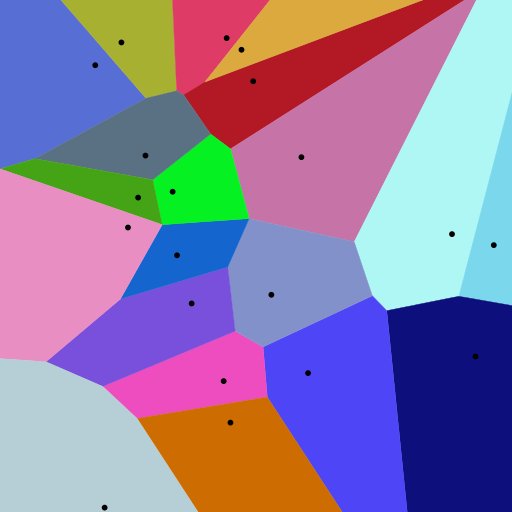## Topics

March 1, 2021

Proximity analysis is one of the cornerstones of spatial analysis. It refers to the ways in which we use spatial methods to ask "what is happening near here". It is at the heart of the Tobler's first law of geography which I paraphrase as "Everything is related but near things are more related." In practice, proximity analysis is often implemented with buffer polygons. A buffer polygon delineates an area or area within a defined radius of a feature of interest. This necessitates the definition of the buffer distance and a clear explanation of why that distance was selected. Another tool for exploring proximity is Voronoi diagram, which is also called Voronoi tessellation, a Voronoi decomposition, a Voronoi partition, or a Dirichlet tessellation.  A Voronoi diagram partitions the area around a set of features into regions where each location within the region is closer to the contained feature than to any other feature. The resultant polygons, called Thiessen polygons, are shown in the image below.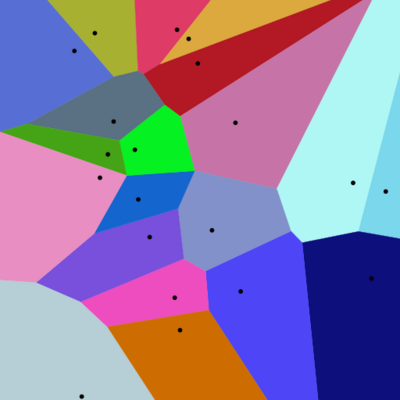Let's take a look at some of the many ways in which proximity analysis can be implemented in R. Keep in mind that this is only an informational example and not a real analysis workflow which would be more complex.

### OVERVIEW

In this example we look at service areas around hospitals in Alameda County, CA. We use the California Healthcare Facility Listing data that we downloaded from the CA Health & Human Services Open Data Portal. For geographic context, we download the 2019 USA counties cartographic boundary file from the Census website and subset to get the border of Alameda County. We use buffers and Voronoi polygons to model the service area for each hospital, considering distance alone. Then we vary these approaches by weighting the analysis based on the number of available hospital beds.

### GETTING STARTED

First, let’s load the R libraries we will use. I encourage you to look at the online documentation for each of these important R spatial libraries.

`library(sf)`

`library(data.table)`

`library(raster)`

`library(rgeos)`

`library(tmap)`

`library(classInt)`

Don’t forget to set your working directory to the folder in which your data resides!

`# Set working directory`

`setwd("~/Documents/proximity_analysis")`

Let’s identify our study polygon as Alameda County, CA. We will load the USA County boundary data downloaded from the Census and then subset to keep just Alameda County.

`# Census USA Counties geography data, cartographic boundary file`

`poly_file <- "cb_2019_us_county_500k.shp"`

`polys <- st_read(poly_file)`

`# Subset County Polygons to Keep only Alameda County`

`my_poly <- polys[polys\$STATEFP=='06'& polys\$COUNTYFP=='001',]`

`# Map it!`

`plot(my_poly\$geometry)`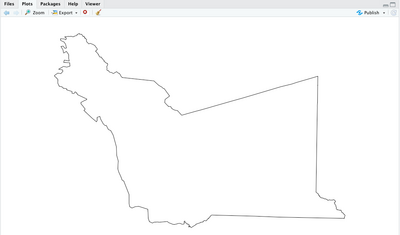We will need to transform all the spatial data we use in this analysis to a planar (2-dimensional) coordinate reference system. This allows our spatial methods to use Euclidean geometry operations, which is required by the libraries we are using. Here we choose the CA Albers coordinate reference system (CRS) as it is appropriate for area analysis within the state of CA.

`# Transform the data to CA ALbers, using the identifier EPSG code 3310`

`my_crs_code <- 3310`

`my_poly <- st_transform(my_poly, crs=my_crs_code)`

Now we are ready to read in the healthcare facility data. We will subset it to keep only hospitals, i.e., where the type is "General Acute Care Hospital" and the number of beds in the facility is greater than 1 (to remove administrative offices).

`# CA Hospital facility points`

`points_file <- "data_chhs_ca_gov_lic_heathcare_fac_20210215.csv"`

`pts_df <- read.csv(points_file)`

`head(pts_df)`

`# keep only facilities with hospital beds`

`nrow(pts_df)`

`pts_df <- pts_df[pts_df\$TOTAL_NUMBER_BEDS > 1,]`

`nrow(pts_df)`

`# and where the type of facility is equal to "General Acute Care Hospital"`

`unique(pts_df\$LICENSE_CATEGORY_DESC)`

`pts_df <- pts_df[pts_df\$LICENSE_CATEGORY_DESC=="General Acute Care Hospital",]`

`nrow(pts_df)`

The hospital data are originally in a CSV file and we read them into an R dataframe. In order to make these data spatial points we need to convert them using the sf library’s function st_as_sf which in this case returns a SpatialPointsDataframe. The inputs to this function are (1) the name of the dataframe (2) the columns in that dataframe that contain the x and y coordinate data (in that order), and the CRS of the data as it is encoded in the dataframe. We then transform the data to CA Albers to use in our analysis.

`# make dataframe an sf::SpatialPointsDataFrame`

`pts = st_as_sf(pts_df,`

`                coords = c('LONGITUDE', 'LATITUDE'),`

`                crs = 4326)`

`plot(pts\$geometry)`

`# transform the CRS to CA Albers`

`my_pts <- st_transform(pts, crs=my_crs_code)`

`# Subset - select only those points in Alameda`

`my_pts <- st_intersection(my_pts, my_poly)`

`# Map the points on top of Alameda County`

`plot(my_poly\$geometry)`

`plot(my_pts\$geometry, add=T`)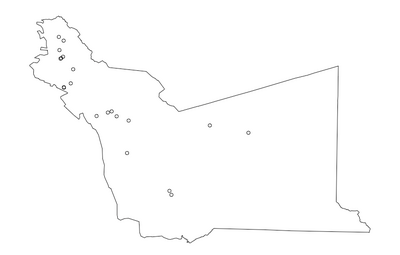### BUFFER ANALYSIS

The sf library makes it relatively straightforward to create distance based buffers with the st_buffer operation where the two key imports are the name of the sf object with the features around which to buffer and the buffer distance in the units of the CRS of the sf object. For the CA Albers CRS the units are meters.  In the code below we define a buffer distance of 2000 meters, or 2km. This will define areas that are 2KM around each point. We may wish to do this if our assumption is that people use the closest hospital if it is within 2KM. We know that to be an oversimplification!

`# Identify buffer distance in units of the CRS, here meters`

`bufdist <- 2000  # or 2km`

`# Implement the buffer operation on the points`

`# which outputs polygons for each point`

`pts_buf <- st_buffer(my_pts, dist=bufdist)`

`# Plot results`

`plot(my_poly\$geometry)`

`plot(pts_buf\$geometry, add=T)`

`plot(my_pts\$geometry, col="red", add=T)`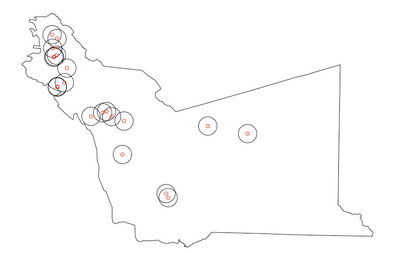Now those buffers are all the same size regardless of the number of hospital beds. So let’s vary the size of the buffers based on the number of beds. The way we do this is as follows.

• First we use the classInt::classIntervals function to create a set of break points based on binning the points into 5 quantiles based on the number of beds.
• We then use those class breaks to create a bed_weight variable that ranges from 1-5 for each quantile.
• We set the initial buffer size to 1000 meters and then multiply it by the bed_weight to get a buffer distance that ranges from 1 to 5 KM.

`# Take a look at the range of values for number of beds`

`summary(as.numeric(my_pts\$TOTAL_NUMBER_BEDS))`

`# make the number of beds an integer variable`

`my_pts\$TOTAL_NUMBER_BEDS <- as.numeric(my_pts\$TOTAL_NUMBER_BEDS)`

`# Now lets use the classInt package to classify the number of beds into weights`

`# depending on what quantile they fall into`

`my_cats <- classIntervals(my_pts\$TOTAL_NUMBER_BEDS, n=5, style="quantile")`

`my_cats`

`names(my_cats)`

`# Make a weights vector that we can add back to the dataframe`

`my_weights <- cut(my_pts\$TOTAL_NUMBER_BEDS, breaks = my_cats\$brks, labels=as.numeric(1:5))`

`length(my_weights)`

`nrow(my_pts)`

`my_pts\$bed_weights <- cut(my_pts\$TOTAL_NUMBER_BEDS, breaks = my_cats\$brks, labels=F)`

`#Check the shape of the bed_weights var`

`summary(my_pts\$bed_weights)`

`# if any weights are NA set them to one`

`my_pts\$bed_weights[is.na(my_pts\$bed_weights)] <- 1`

`# Now create a buffer that multiplies our base distance times the weight`

`bufdist = 1000`

`pts_bufw <- st_buffer(my_pts, (bufdist*my_pts\$bed_weights))`

`#finally, map the output`

`plot(my_poly\$geometry)`

`plot(pts_bufw\$geometry, add=T)`

`plot(my_pts\$geometry, col="red", add=T)`Our variable distance polygons take into account the ability of a hospital with a lot of beds to service a larger area.

### VORONOI DIAGRAM

Our buffer polygons do not account for all of the area around a hospital, e.g., if an area isn’t in the buffer it is not assigned to a hospital. But we know that everyone may need to go to a hospital at some point. So let’s consider how a Voronoi diagram can be used to model proximity.

In the code below, after that from Dan Houghton on github, we partition all of Alameda County so that each area within it is assigned to the nearest hospital.

`voronoi <- `

`  my_pts %>% `

`  st_geometry() %>%`

`  st_union() %>%`

`  st_voronoi() %>%`

`  st_collection_extract()`

`# Put output back in their original order`

`# so can easily rejoin with source attributes`

`voronoi <-`

`  voronoi[unlist(st_intersects(my_pts,voronoi))]`

`# Plot the point voronoi polygons`

`plot(voronoi)`

`# clip the voronoi polys to the boundary`

`voronoi2 <- st_intersection(my_poly\$geometry, voronoi)`

`# plot the clipped voronoi polygons`

`plot(voronoi2)`

`plot(my_pts\$geometry, add=T, col="red", pch=20)`

`# Make the voronoi polygon data an sf object`

`# that has the voronoi geometry but the input attributes`

`voronoi_sf = st_sf(my_pts, geometry = voronoi2)    # sf object`

`st_set_crs(voronoi_sf, my_crs_code)`

`st_crs(voronoi_sf)`

`# Plot it to check`

`plot(voronoi_sf\$geometry)`

`plot(my_pts\$geometry, add=T, col="red", pch=20)`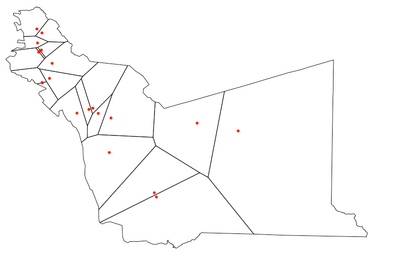COMBINING BUFFERS AND VORONOI POLYGONS

We can combine these two approaches as a way of implementing the assumption that an area will be served by the closest hospital, but only within a specific distance.

Here we implement this combining Voronoi polygons clipped to fixed distance buffers, again following code by Dan Houghton:

`st_buffer_without_overlap <- function(centroids, dist) {`

`  # Voronoi tesselation`

`  voronoi <- `

`    centroids %>% `

`    st_geometry() %>%`

`    st_union() %>%`

`    st_voronoi() %>%`

`    st_collection_extract()`

`  # Put them back in their original order`

`  voronoi <-`

`    voronoi[unlist(st_intersects(centroids,voronoi))]`

`  # Keep the attributes`

`  result <- centroids`

`  # Intersect voronoi zones with buffer zones`

`  st_geometry(result) <-`

`    mapply(function(x,y) st_intersection(x,y),`

`           st_buffer(st_geometry(centroids),dist), `

`           voronoi,`

`           SIMPLIFY=FALSE) %>%`

`    st_sfc(crs=st_crs(centroids))`

`  result`

`}`

`buffer_distance_meters <- 2000 # 2000 meters or 2 km`

`pts_buffered <- st_buffer_without_overlap(my_pts, buffer_distance_meters)`

`# Plot buffer points`

`plot(my_poly\$geometry)`

`plot(pts_buffered\$geometry, add=T)`

`# Clip buffer points`

`pts_buffered_clipped <- st_intersection(my_poly, pts_buffered)`

`# plot clipped points`

`plot(my_poly\$geometry) `

`plot(pts_buffered_clipped, col="beige", add=T)`

`plot(my_pts\$geometry, pch=20, col="red", add=T)`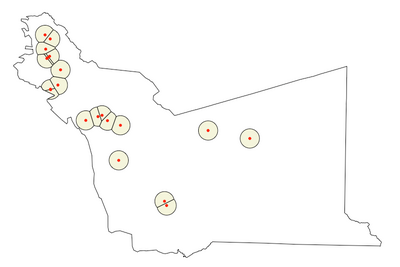Here we implement same approach with with variable width buffers

`st_buffer_without_overlap_weighted <- function(centroids, dist, weight_var) {`

`  # Voronoi tesselation`

`  voronoi <- `

`    centroids %>% `

`    st_geometry() %>%`

`    st_union() %>%`

`    st_voronoi() %>%`

`    st_collection_extract()`

`  # Put them back in their original order`

`  voronoi <-`

`    voronoi[unlist(st_intersects(centroids,voronoi))]`

`  # Keep the attributes`

`  result <- centroids`

`  # Intersect voronoi zones with buffer zones`

`  st_geometry(result) <-`

`    mapply(function(x,y) st_intersection(x,y),`

`           st_buffer(st_geometry(centroids), (dist*centroids[[weight_var]])), `

`           voronoi,`

`           SIMPLIFY=FALSE) %>%`

`    st_sfc(crs=st_crs(centroids))`

`  result`

`}`

`#`

`buffer_distance_meters <- 1000 # 20000 meters or 20 km`

`pts_buffered2 <- st_buffer_without_overlap_weighted(my_pts, buffer_distance_meters, 'bed_weights')`

`# Plot buffer points`

`plot(my_pts\$geometry)`

`plot(pts_buffered2\$geometry, add=T)`

`# clip `

`pts_buffered_clipped2 <- st_intersection(my_poly, pts_buffered2)`

`# plot clipped points`

`plot(my_poly\$geometry) `

`plot(pts_buffered_clipped2, col="beige", add=T)`

`plot(my_pts\$geometry, pch=20, col="red", add=T)`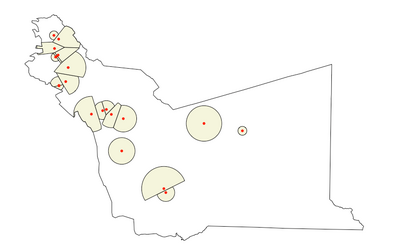WEIGHTED VORONOI DIAGRAM

For our final example, we are going to implement code to create a weighted Voronoi diagram as a way to partition the study area but giving more weight to hospitals with a larger bed capacity. This code is borrowed from two Stack Overflow posts, one by Dr. William Huber that describes Thiessen polygons in detail, and one by Simone Bianchi who provide code for how to implement their creation in R. I recommend that you read both of these posts. I provide the code here so that you can see how to implement it with sf objects in R using data that is available online. In this example, we use the bed weights to multiplicatively vary the size of the Voronoi polygons around each point. Following Bianchi, we implement a raster implementation due to the computation complexity of generating a vector weighted Voronoi partition.  This assumes some knowledge of raster data structures and methods in R.

`################`

`# weighted voronoi`

`################`

`rez= 1000 # this number is the cell size and depends on the size of the study area`

`maxlen=603 # WHERE DID 603 come from? It should be the distance / rez`

`my_id_var <- 'OSHPD_ID'`

`my_weight_var <- 'bed_weights'`

`r <- raster(xmn=sf::st_bbox(my_poly)[['xmin']], `

`            xmx=(sf::st_bbox(my_poly)[['xmin']] + (maxlen*rez)), `

`            ymn=sf::st_bbox(my_poly)[['ymin']], `

`            ymx=(sf::st_bbox(my_poly)[['ymin']] + (maxlen*rez)), `

`            crs = sf::st_crs(my_poly)\$proj4string, resolution=rez)`

`pts_dt <- data.table(`

`  ptID = paste0('x',my_pts[[my_id_var]]),  # ID MUST BE A STR and UNIQUE`

`  x = st_coordinates(my_pts)[,1] ,`

`  y = st_coordinates(my_pts)[,2],`

`  size=my_pts[[my_weight_var]]`

`)`

`head(pts_dt)`

`# create an empty datatable with the length equal to the raster size`

`dt <- data.table(V1=seq(1, length.out = (maxlen*rez)^2*(1/rez)^2))`

`# calculate distance grid for each tree, apply weight and store the data`

`for (i in pts_dt[, unique(ptID)]){`

`  #print(i)`

`  # 1) standard Euclidean distance between each cell and each point`

`  d <- distanceFromPoints(r, pts_dt[ptID==i,.(x,y)])`

`  # 2) apply your own custom weight`

`  # modify distance values by point weight (should set weight default to 1)`

`  d <- ((d@data@values)^2)/pts_dt[ptID==i,size]`

`  # 3) store the results with the correct name`

`  dt <- cbind(dt,d)`

`  #print(i)`

`  setnames(dt, "d", i)`

`}`

`# carry out comparison`

`# find the column with the lowest value (+1 to account to column V1 at the beginning)`

`# ie. find the smallest distance between a cell and a point`

`dt2<-dt`

`dt[,minval:=which.min(.SD)+1, by=V1, .SDcols=2:length(names(dt))]`

`# find the name of that column, that is the id of the point`

`dt[,minlab:=names(dt)[minval]]`

`# create resulting raster`

`out <- raster(xmn=sf::st_bbox(my_poly)[['xmin']], `

`              xmx=(sf::st_bbox(my_poly)[['xmin']] + (maxlen*rez)), `

`              ymn=sf::st_bbox(my_poly)[['ymin']], `

`              ymx=(sf::st_bbox(my_poly)[['ymin']] + (maxlen*rez)), `

`              crs = sf::st_crs(my_poly)\$proj4string, resolution=rez,`

`              vals=dt[,as.factor(minlab)]`

`)`

`plot(out)`

`out_masked <- mask(out, my_poly)`

`plot(out_masked)`

`plot(my_poly, add=T)`

`# Transform into polygons`

`# Note outpt is class sp`

`outpoly <- rasterToPolygons(out_masked, dissolve=T)`

`outpoly_sf <-st_as_sf(outpoly)`

`# add the point ids to the polys`

`x <- dt[,as.factor(minlab)]`

`outpoly_sf\$nearpt_id <- levels(x)[outpoly_sf\$layer]`

`# Plot results with starting points`

`plot(outpoly_sf\$geometry, col="beige");`

`plot(my_pts\$geometry, col="red",add=T)`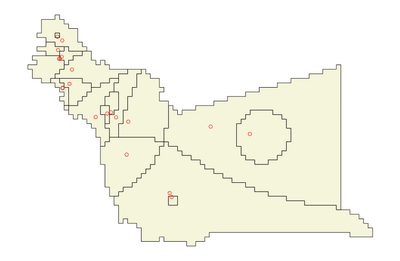Voronoi and Weighted Voronoi polygons partition space into neighborhoods with similar estimated characteristics, hospital service areas in this example. As such, Voronoi diagrams are a method of spatial interpolation. These polygons can also serve as inputs to adjacency based methods of proximity analysis such as spatial autocorrelation, which considers patterns and trends in the data. Spatial analysis provides many ways to consider neighborliness.

### CONCLUDING THOUGHTS

That’s a lot of proximity analysis code to chew on! I hope that there is some useful code in this post, whatever type of neighborhood you are trying to model.  I also hope you can see from this post just how powerful R is as a computing environment for spatial analysis. The R sf and raster packages and related libraries provide a fantastic complement to desktop GIS software like ArcGIS and QGIS.  If you want to learn more, register for the D-Lab’s R Geospatial Data Fundamentals workshop, one iteration of which will be held in March 2021. I also strongly (and often) recommend the great online book Geocomputation with R by Robin Lovelace, Jakub Nowosad, and Jannes Muenchow for those interested in geospatial data and analysis in R.